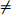Hello, dear friend, you can consult us at any time if you have any questions, add WeChat: daixieit

Math 240 Midterm Review

1. (Propositional Logic) Simplify the following statements. Your final answers should use only the symbols p, q, r, ¬, ∨, ∧.

(a) (p ⇒ q) ∧ (q ⇒ ¬p)

(b) (p ⇔ q) ∨ (p ⇔ ¬q)

(c) (p ∨ q) ∧ (p ∨ r) ∧ ¬(q ∧ r)

2. (Quantifiers) Write the negation of each of the following statements. In each case, determine whether the original or the negation is true.

(a) (In the universe of P(N)) ∀X∃Y ( (X ∩ Y = ∅) ∧ (X ∪ Y = N))

(b) (In the universe of Z) ∀x∃y∀z(yz2 < x)

(c) (In the universe of Z) ∀x∃y( (x < 0) ∨ (x = y2))

3. (Sets) Let A, B be sets.

(a) Prove that (A ∩ B) × B ⊆ B × B.

(b) Prove that A × B ⊆ A × (A ∪ B).

(c) Suppose that A ∪ B = B. Which of the following is true:

i. A = B.

ii. A ⊆ B.

iii. B ⊆ A.

4. (Functions) Determine whether the following functions are injective/surjective/bijective.

(a) f : Z × Z → Z defined by

f(a, b) = ab

(b) f : R → Z defined by

f(x) =xRecall the floor functionxwhich gives the largest integer less than or equal to x. For example:2.9= 2,π= 3,−5.5= −6.

(c) f : P(N) × P(N) → P(N) defined by

f(X, Y) = X ∩ Y

5. (Proofs)

(a) Prove the following statement: If a and b are rational then a + b is rational.

(b) Prove the following statement: If a is rational and b is irrational then a + b is irrational.

(c) Consider the similar statement: If a and b are irrational then a + b is irrational. Is this statement true?

(d) Prove the following statement: If a is rational then a2 + a + 10.

6. (Relations) For each of the following relations, determine whether the relation is reflexive/symmetric/transitive (assume the universe is R).

(a) aRb if ab.

(b) aRb if ab0.

(c) aRb if 5|(b − a).

(d) aRb if a = b + 1.

(e) aRb if a + b = 0.